## Mastering Important Concept Test by GMAT in Triangle - III

We invite all community contributors to share resources that are deemed to help students beat the GMAT. We've created individual threads to match the main topics, so please select the best fit for your content carefully.

### GMAT/MBA Expert

GMAT Instructor
Posts: 645
Joined: 26 Jul 2010
Location: US
Thanked: 527 times
Followed by:227 members

### Mastering Important Concept Test by GMAT in Triangle - III

by e-GMAT » Mon Dec 12, 2016 12:38 am
Added the PDF of the article at the end of the post!Mastering Important Concept Tested by GMAT on Triangles - III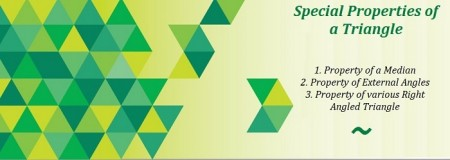This is the third and final article in our series of articles on Triangles. In case you haven't gone through the previous articles, we strongly suggest that you go through them before reading this article. The previous articles can be accessed using the following links:

In the above articles, we have discussed the following aspects:
• 1. Identifying whether a set of given numbers could be the lengths of the three sides of a triangle.
2. Range of possible values of the length of one side of a triangle given the lengths of the other two sides.
3. Key properties related to the angles of a triangle.
4. Key properties of different types of triangles tested on the GMAT.

Carrying on with the discussion, let's now look at the last article on Triangles, where 3 special properties will be discussed which are tested by GMAT.

We will also apply the learning from this article on three GMAT like questions to understand the application of the concepts.

1. LEARN HOW A MEDIAN AFFECTS THE AREA OF ANY TRIANGLE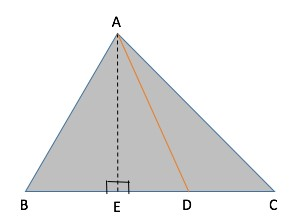Consider any triangle ABC and draw a line joining A to the midpoint of BC. Let's call this line AD.
Now tell me, what can we infer about the area of triangle ABD and ACD? Is there any relation between them?

We know, Area of a triangle = (1/2) * base * height

So, let us drop a perpendicular from A on BC and name it AE. Since the heights of triangle ABD and ACD are equal to AE, we can write:
"¢ Area of triangle ABD = Â½ * BD * AE and Area of triangle ACD = Â½ * CD * AE

Also, since D is the midpoint of BC, we have CD = BD . Hence, we can conclude that:
Area of triangle ABD = Area of triangle ACD.

We know that the line joining a vertex of a triangle to the midpoint of the opposite side is called a median of the triangle.

Therefore, we found that the median AD divides the triangle into two equal areas.

Let us look at an Official question which can be solved very quickly, using the above property.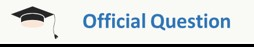Property of a Median playing an important role in a OG Question. A slightly edited version of OG Question: OG 16 DS 126In triangle ABC, point X is the midpoint of side AC and point Y is the midpoint of side BC. If point R is the midpoint of line segment XC and if point S is the midpoint of line segment YC, what is the area of triangular region RCS, if the area of triangular region ABX is 32 square units?

This diagram might look scary and the question might seem undoable at the beginning. But let us simplify the diagram, concentrating only on the medians, BX, XY, RS and RY (join RY in the diagram). On doing so, the diagram will look as follows: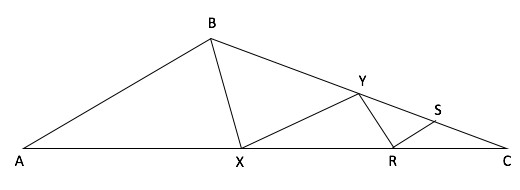Solution:
Since BX is a median in triangle ABC, we can use the Special Property to conclude that, Area of ABX = Area of BXC
It is given that the area of triangle ABX = 32 square units. Hence, Area of BXC = 32 square units.

Since XY is a median in triangle BXC, we can conclude that
"¢ Area of BXY = Area of XYC = 32/2 = 16

Since RY is a median in triangle XYC, we can conclude that
"¢ Area of RYX = Area of RYC = 16/2 = 8

And since RS is a median in triangle YRC we can conclude that
"¢ Area of YRS = Area of RCS = 8/2 = 4
So, is this question really tough? Definitely, not as tough as it looked at the beginning. We used one simple and special property to elegantly solve a complicated looking question.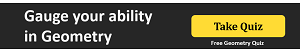2. DISCOVER THE PROPERTY OF SUM OF EXTERNAL ANGLES OF A TRIANGLE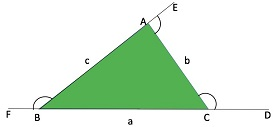We know that, an external angle = sum of two opposite interior angles. In the above diagram, ABC is a triangle with sides extended as shown. Therefore, using the above property we can write,

Ext. Angle ACD = int. angle CAB + int. angle CBA

But hold on, that is not the special property! Now you know that the sum of internal angles = 180 .
But what about the sum of external angles? If we add up all the external angles as shown in the diagram, we will get

Ext Angles (ACD + CAE + ABF) = int. angles (2A + 2B + 2C) = 2 * 180 = 360

Hence, the sum of external angles = 360 .

We will discuss this property in more details for Polygons, where I will show you that sum of external angles is equal to 360 for any convex polygon, irrespective of the number of sides the polygon has.

3. CHEEKY TRICK ON ANGLE-SIDE PROPERTY OF SPECIAL RIGHT ANGLED TRIANGLES

While going through GMAT questions on right angled triangles, the most commonly tested right angled triangles are ones with the following set of values as its angles.
1. 30 - 60 - 90 or 2. 45 - 45 - 90 .

Therefore, it important for us to know the relation between these angles and the sides of the triangles, as knowing this can help us in finding the answer quickly and at the same make the calculations significantly simple.

Case 1: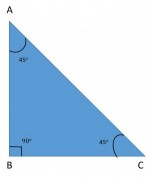As shown in the figure, if the angles of the triangle are 30 - 60 - 90 , the ratio of the sides will be -

AB: BC: AC = 1: âˆš3: 2

Case 2:1. As shown in the figure, if the angles of the triangle are 45 - 45 - 90 , the ratio of the sides will be -

AB: BC: AC = 1: 1: âˆš2

Note: The above ratio can easily be proved using Trigonometry values.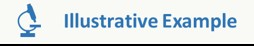Application of Special Properties on a question similar to OG16 -PS214

Let us apply the special property 2 and 3 in a very simple GMAT like question. The two questions given below are based on the same diagram, so lets solve these questions together to understand the application of the above properties.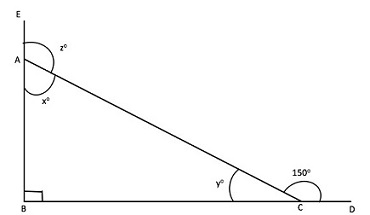Q.1 What is the value of z?
• a. 110
b. 120
c. 130
d. 140
e. 150
Q.2 If the value of AC = 4 units , what is the ratio of the sides AB and BC ?
• a. 2 : 1
b. 1 : âˆš3
c. 2 : âˆš3
d. âˆš3 : 1
e. 1 : 2
Solution to Q1:
Notice carefully, that z is the external angle of triangle ABC. From our conceptual understanding related to angles of a triangle, we can write -
• "¢ External Angle CAE = sum of opposite internal angles of triangle ABC
"¢ Therefore, Angle z = Angle ABC + Angle ACB
From the diagram, we can clearly infer that -
• "¢ Angle ABC = 90 and Angle ACB = y .
Also,
• "¢ Angle ACB + Angle ACD = 180 , [straight line angle property]
• "¢ Angle ACD = 150
• o Therefore, Angle ACB = 180 - 150 = 30 .
Hence, the value of Angle z = Angle ABC + Angle ACB = 90 + 30 = 120 .

Solution to Q2:
This question can be solved in multiple ways -

1. Method - I:
We know that AC = 4 units , and from our above discussion in part 1 we know that angle ACB = 30 . [/list]
Therefore,
• o length AB = AC sin 30= 4 * Â½ = 2
o And BC = AC cos 30= 4 * âˆš3/2 = 2âˆš3
Therefore, ratio of AB : BC = 2 : 2âˆš3 = 1 : âˆš3

2. Method - II:
But say one does not know trigonometry but still wants to solve it. This is where, our Special Property 3, Case 1 comes in handy!
• o If we observe carefully, we will notice that triangle ABC is a 30 - 60- 90triangle.
o Since ABC = 90 , BAC = 60 and ACB = 30
o And we know that ratio of AB : BC : CA = 1 : âˆš3 : 2
Therefore, from here, we can directly say that the answer would be 1 : âˆš3 and we don't even have to use the value of AC to find the answer!

Cool isn't it?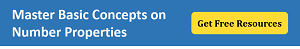Now, let us come to the last example of our article, where we will try to apply the third property in a question, which not only focuses our conceptual knowledge but also tests are ability to represent the information in a diagrammatically correct manner.A question to test your imagination!!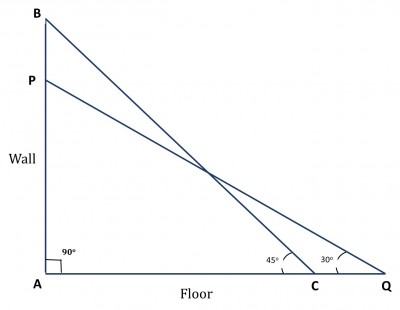A 60 feet long ladder is inclined against a wall such that the bottom and the top of the ladder are at equal distance from the point where the wall meets the floor. The same ladder slides away from the foot of the wall such that it is inclined at an angle of 30 from the floor. What is the difference between the heights to which the ladder reaches in the two cases?
• A. 15(âˆš2 - 1)
B. 30(âˆš2 - 1)
C. 15(âˆš2 + 1)
D. 30âˆš2
E. 30(âˆš2 + 1)
Solution:
Now this question might seem to be a tough one, because the position of the ladder is different in the two cases, which leads to change in the angles with respect to the floor. Therefore, most students might feel that this question might not be an easy one to solve.

But no need to worry, let's see how by using our special property and a methodical approach, we can get our answer very easily!

Step 1: Drawing Inferences from the Question Statement
Let us represent the two cases diagrammatically.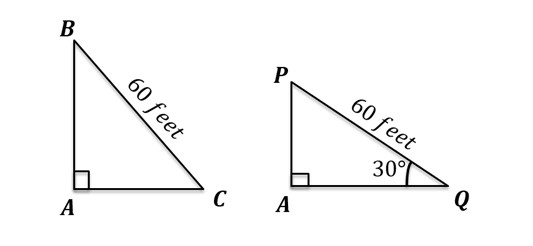Triangle BAC represents the initial case, where point A is the point of intersection of the wall and the floor and BC represents the ladder. We are given that AB = AC and BC = 60 feet.
Triangle PAQ represents the case after the ladder slided. The only value that remains unchanged is the length of the ladder, hence BC = PQ.

Therefore, in the Right Triangle PAQ, PQ = 60 feet , and Angle AQP = 30
We need to find the value of AB - AP.

Step 2: Finding required values

Let us first consider Right Triangle BAC:
We know that when two sides of a right triangle are equal, it is 45 - 45 - 90triangle.
As discussed in special property 3, in such a triangle, the sides opposite to the angles 45 , 45 , and 90 respectively are in the ratio 1: 1: âˆš2
Therefore,
AB/BC=1/(âˆš2)
Or, AB=BC/âˆš2=60/âˆš2=30âˆš2
Thus, we have found out the value of AB.
Now, considering Right Triangle PAQ:
It is 30 - 60 - 90 right angled triangle.

And we know that in such a triangle, the sides opposite to the angles 30 , 60 , and 90 respectively are in the ratio 1 : âˆš3 : 2.
Therefore, AP/PQ=1/2
Or, AP=PQ/2=60/2=30

Step 3: Calculate the final answer
Difference between the heights to which the ladder reaches in the two cases = AB - AP = 30âˆš2 - 30 = 30(âˆš2 - 1)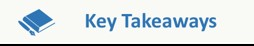What did we learn???

Any geometry question testing the concepts of triangles can be easily tackled as long as the test taker is clear with:
• a. the basic properties of a triangle
b. the subtle differences these properties exhibit, depending on the type of the triangle
c. when to apply and how to apply these basic properties.
Practice questions will be posted in a few days to test your understanding of all the concepts that we have discussed in our three articles. Feel free to post any question till then.Attachments
Triangle_Part3_BTG.pdf